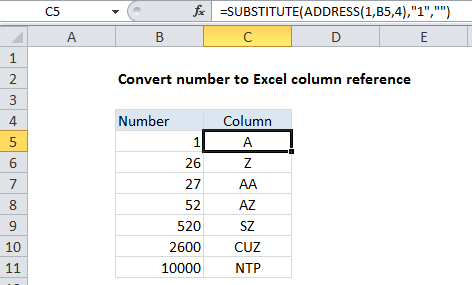## Excel Office

Excel How Tos, Tutorials, Tips & Tricks, Shortcuts

# Convert column number to letter in Excel

To convert a column number to an Excel column letter (e.g. A, B, C, etc.) you can use a formula based on the ADDRESS and SUBSTITUTE functions. See example below:

## Formula

`=SUBSTITUTE(ADDRESS(1,col_number,4),"1","")`## Explanation

In the example shown, the formula in C5 is:

`=SUBSTITUTE(ADDRESS(1,B5,4),"1","")`

The first step is to construct an address that contains the column number. We do this with the ADDRESS function, by providing 1 for row number, a column number from B5, and 4 for the abs_num argument (to get a relative reference):

`ADDRESS(1,B5,4)`

With this information, ADDRESS returns the text “A1”.

Worked Example:   Manipulating text strings using Left, Mid, Right, Len, Substitute in Excel

Next ,we use SUBSTITUTE to strip out the number 1 like this:

`=SUBSTITUTE("A1","1","")`

We can confidently look for “1” and replace with “” in all cases, because the row number is hard-coded as 1 inside the ADDRESS function.

Worked Example:   Remove text by matching in a cell in Excel